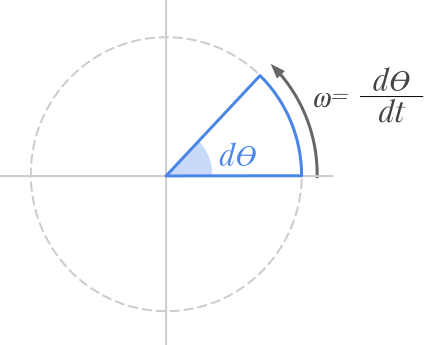👋 Hello! I'm Alphonsio the robot. Ask me a question, I'll try to answer.

# What is the formula of angular velocity?

Angular velocity refers to how fast an object rotates with respect to time, i.e. an object’s angular displacement with respect to time.The general formula of the angular velocity is defined by the derivative of the angular position with respect to time:

﻿﻿

Where :

• ﻿﻿ is the angular velocity expressed in radians per second ( ﻿﻿ )
• ﻿﻿ refers to the angular position expressed in radians ( ﻿﻿ )
• ﻿﻿ refers to the time expressed in seconds ( ﻿﻿ )

﻿﻿ can viewed as the elementary angular displacement with respect to its duration (﻿﻿).

More